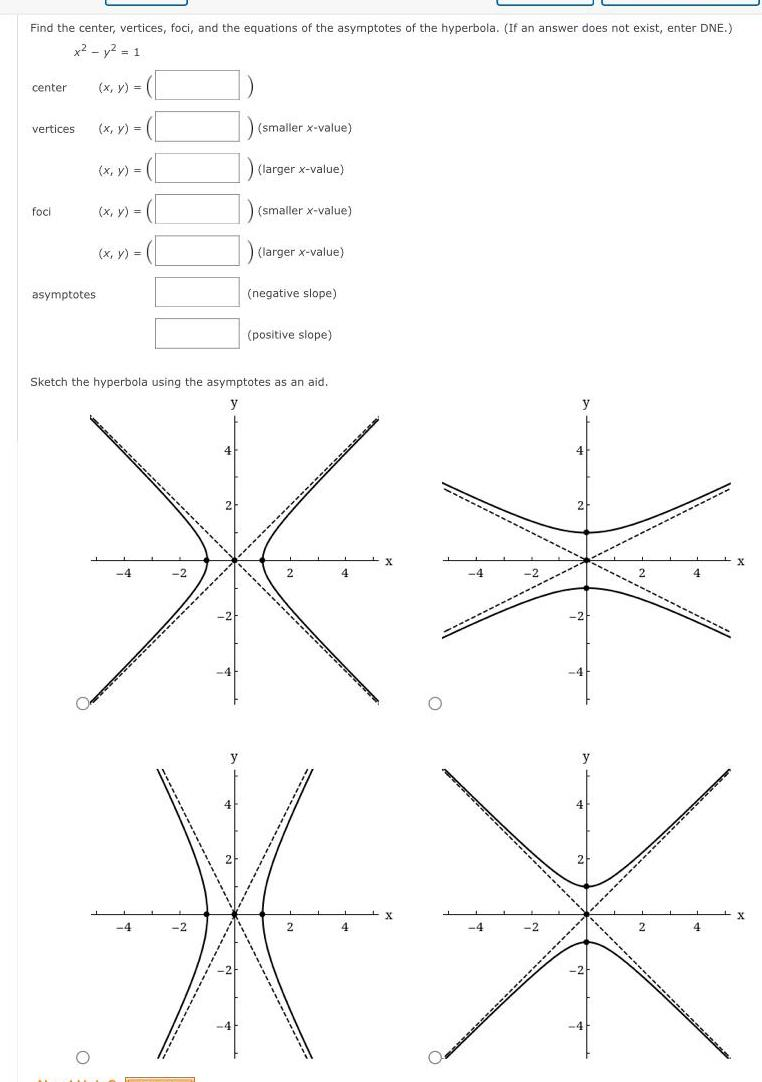Question:

# Find the center vertices foci and the equations of the

Last updated: 5/11/2023Find the center vertices foci and the equations of the asymptotes of the hyperbola If an answer does not exist enter DNE x y 1 x y center vertices foci asymptotes x y x y x y x y 4 2 smaller x value y larger x value smaller x value larger x value Sketch the hyperbola using the asymptotes as an aid y 4 2 negative slope positive slope 2 4 X erararas 4 2 y 2 2 2 2 4 X X